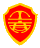`一级建造师二级建造师造价工程师监理工程师房地产估价师土地估价师咨询工程师安全工程师招标师造价员建工网校辅导 7天免费听课建筑标准规范 建筑工艺建筑下载建筑网址大全 选课中心建工网校`
`网校动态网校名师网校课程网校帮助学员心声课件更新免费试听招生方案快速选课  `

(1)材料费=材料净重×(1+加工损耗系数) ×每吨材料综合价

(2)辅助材料费=设备总重量×辅助材料费指标

(3)加 工 费=设备总重量(吨)×设备每吨加工费(4)专用工具费=(材料费+辅助材料费+加工费)×专用工具费率

(5)废品损失费=(材料费+辅助材料费+加工费)×(1+专用工具费率)×废品损失费率

(6)外购配套件费

(7)包装费=[(材料费+辅助材料费+加工费) ×(1+专用工具费率) ×(1+废品损失费率)+外购配套件费] ×包装费率

(8)利润={[(材料费+辅助材料费+加工费) ×(1+专用工具费率) ×(1+废品损失费率)+外购配套件费]×(1+包装费率)-外购配套件费｝×利润率外购配套件费计取包装费，但不计取利润。

(9)税金，主要指增值税，通常是指设备制造厂销售设备时向购入设备方收取的销项税额。

当期销售税额=销售额×适用增值税率销售额={[(材料费+辅助材料费+加工费) ×(1+专用工具费率) ×(1+废品损失费率)+外购配套件费]×(1+包装费率)-外购配套件费｝×(1+利润率)+外购配套件费

(10)非标准设备设计费非标准设备设计费独立计算，与其他 9 项费用无关。

单台非标准设备原价={[(材料费+辅助材料费+加工费) ×(1+专用工具费率) ×(1+废品损失费率)+外购配套件费] ×(1+包装费率)-外购配套件费}×(1+利润率)+外购配套件费+销项税额+非标准设备设计费

tags：国产非标准设备原价

## 相关资讯